## Math 480 Course Notes -- June 12, 1996

### Solution of the simplest stochastic DE model for asset prices; Ito's lemma

The simplest stochastic model for the price of an asset begins with the exponential model and adds a random "volatility" term: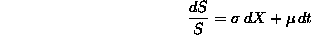In this equation, S(t) is a random variable-valued function of time that represents the price of the asset at time t,is the deterministic rate of growth of the asset price, andis a positive constant that provides a measure of the degree of variation in the price, called "volatility". For most stocks,is between 0.05 and 0.4. The dX in the equation provides the random fluctuations in the asset price. We assume that dX is derived from a Weiner process, i.e.,

1. X(t) is a random variable.
2. For each s and t, X(s)-X(t) is a normally distributed random variable with mean 0 and variance (s-t). (This forces the dX=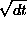condition we encountered before).
3. For s<t<u, the normally distributed random variables X(u)-X(t) and X(t)-X(s) are independent (this is a Markov assumption!).

In order to solve this equation, we recall the solution of the constant coefficient stochastic differential equation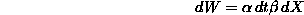that led us earlier to the Fokker-Planck equation. Recall that the solution of this equation for W was a Weiner process with drift coefficientand diffusion coefficient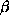, and that if we knew that at t=0 the (deterministic) value of W was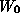, then W(t) is a normally distributed random variable with meanand variance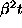. In other words, the pdf of W(t) iswhich satisfies the Fokker-Planck equationFrom the (stochastic differential) equation satisfied by S, it appears that log(S) should satisfy a Fokker-Planck equation. This is essentially true, but to derive the solution of the equation for S, we need one of the most important results in stochastic analysis, namely Ito's Lemma. This lemma is essentially the chain rule for stochastic functions. Before we state it, recall the chain rule for "ordinary" functions (of t):

ORDINARY CHAIN RULE: Let f(t) be a given function of t, with differential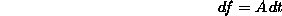Let y=g(f,t) be a function of f and t. Then the differential of g isTo obtain this, we take the differential of g as a function of the two variables f and t, and substitute what we already know about df.

The extra complication that enters with stochastic functions is that each variable can depend upon t and the Wiener process X. Moreover, the dependence on X must be tracked up to second order (i.e., to include both dX and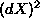terms). Finally, we must keep in mind that for the Wiener process, we have=dt.

ITO'S LEMMA: Let W be the Wiener process given above (with driftand diffusion). Let f(W,t) be a function of W and t. Then the differential of f is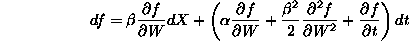You can see the terms from the "ordinary" chain rule, but there are a few extra terms. We justify this as follows:

Because we must keep terms up to order 2 in dX (and thus in dW), we expand f in a Taylor series as follows (we're a little casual with the notation, we write df for the increment in f and don't bother too much with limits. Keep in mind that we intend to let dt approach 0 and dX approaches 0 at the same rate as, so all our omitted terms will approach zero at a faster rate):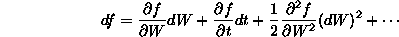Now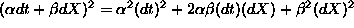. But the first two terms in the expanded square are beyond the order we consider (they go to zero faster than dt), so we can omit them:We substitute this into df: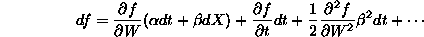In other words,after collecting dt and dX terms. This is Ito's Lemma.

We definitely need an example or two:

First, we derive the formula for the evolution of the price of an asset (more on this later). Because the RELATIVE change in asset prices is more important than their absolute change, a standard assumption is to assume that this relative change follows a Wiener process with a certain drift and diffusion. If S is the asset price, the we assume that(where X is the "standard" Wiener process). In the financial context,is called the growth rate (optimistically) andis called the volatility of the asset.

The left side looks like the differential of log(S) (WARNING: It isn't! The formula dS/S does not take into account the stochastic nature of S!) Therefore, we will attempt to learn about S by calculating the (stochastic) differential of the exponential function of W. With this in mind, let. Then according to Ito's lemma: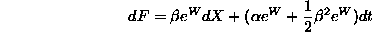In other words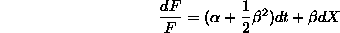This looks like the formula for dS, once we substitute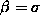and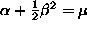. This gives the important result that:

The logarithm of the price of an asset follows a Wiener process (continuous time random walk) with drift coefficientand diffusion coefficient.

This implies that if we know that the asset price at time t=0 is, then the pdf of the asset price S(t) is given by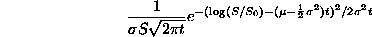Homework

1. Prove this last formula, using the fact that we know the pdf of.

2. Use Ito's lemma to calculate df where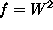. Based on your answer, calculate the value of the stochastic integral:(Hint: the answer is likebut it is a little different!)

Dennis DeTurck
Tue Jun 18 13:40:00 EDT 1996